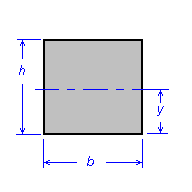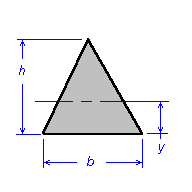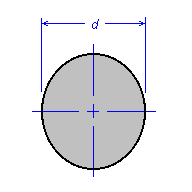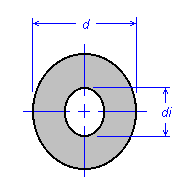# Properties of Sections

This table provides formula for calculating section Area, Moment of inertia, Polar moment of inertia, Section modulus, Radius of gyration, and Centroidal distance, for various cross section shapes.

Formula for rectangular, triangular, hollow circle and full circle sections is provided.

```Nomenclature:

A = Area, in^2

I = Moment of inertia, in^4

J = Polar moment of inertia, in^4

Z = Section modulus, in^3

k = Radius of gyration, in

y = Centroidal distance, inRectangle relationships:

A = b * h

I = b * h^3 / 12

Z = b * h^2 / 6

k = 0.289 * h

y = h / 2

```

```Triangle relationships:

A = b * h / 2

I = b * h^3 / 36

Z = b * h^2 / 24

k = 0.236 * h

y = h / 3

```

```Circle relationships:

A = 3.14159 * d^2 / 4

I = 3.14159 * d^4 / 64

Z = 3.14159 * d^3 / 32

J = 3.14159 * d^4 / 32

k = d / 4

y = d / 2

```

```Hollow circle relationships:

A = 3.14159 / 4 * (d^2 - di^2)

I = 3.14159 / 64 * (d^4 - di^4)

Z = 3.14159 / (32 * d) * (d^4 - di^4)

J = 3.14159 / 32 * (d^4 - di^4)

k = sqrt((d^2 + di^2) / 16)

y = d / 2

```